• 求解一元高次多项式方程的所有实数根的算法 一元高次多项式的形式可以表示为： f(x)=c0+c1x+c2x2+c3x3+...+cnxn=0 f(x) =c _0+c_1x+c_2x^2+c_3x^3+...+c_nx^n=0 f(x)=c0​+c1​x+c2​x2+c3​x3+...+cn​xn=0 ...
求解一元高次多项式方程的所有实数根的算法
一元高次多项式的形式可以表示为：

f

(

x

)

=

c

0

+

c

1

x

+

c

2

x

2

+

c

3

x

3

+

.

.

.

+

c

n

x

n

=

0

f(x) =c _0+c_1x+c_2x^2+c_3x^3+...+c_nx^n=0

一般来说，一元高次多项式方程很难有显示解析解，大多使用数值算法计算方程的根，例如牛顿法、割线法等。但这些算法的缺点是计算得到的解受到迭代初始值的影响，另外在某些特定情况下，迭代也有可能不收敛。对于拥有多个实数根的多项式方程，使用这些迭代算法难以获得方程所有实数根。 本文将介绍一种新的算法，通过递归降次，将高次多项式方程的求解问题逐步转化为可以解析求解的一元二次方程，进而求解出多项式的所有实数根。
原理
首先，为了求解多项式方程的所有实根，我们需要对多项式函数进行分段。使用

x

1

,

x

2

,

x

3

,

.

.

.

,

x

k

x_1,x_2,x_3,...,x_k

这一系列的点将实数轴分成若干区间段，以使得在每一个区间段上函数f(x)都是单调的。 当我们获得了这一系列的分隔点后，例如上述k个分隔点，将实数轴分为k+1个区间段，（包括

(

−

∞

,

x

1

]

(-\infin,x_1]

和

[

x

k

,

+

∞

)

[x_k,+\infin)

两个区间）。在每一个区间中函数都是单调的。对于区间

[

x

i

,

x

i

+

1

]

[x_i,x_{i+1}]

，如果

f

(

x

i

)

f(x_i)

和

f

(

x

i

+

1

)

f(x_{i+1})

符号相反，由于函数在区间中是单调的，在该区间中必然有且仅有一个实根。这时只要我们在该区间中使用最简单的二分法就能得到该区间中的或者一个实根。 当然，如果

f

(

x

i

)

f(x_i)

和

f

(

x

i

+

1

)

f(x_{i+1})

符号相同，由于函数单调，在该区间中必然不存在方程的实根。 对于

(

−

∞

,

x

1

]

(-\infin,x_1]

这个区间，可以先算出

f

(

x

1

)

f(x_1)

和

f

(

x

1

−

1

)

f(x_1-1)

，如果

f

(

x

1

−

1

)

f(x_1-1)

和

f

(

x

1

)

f(x_1)

同号，且

f

(

x

1

−

1

)

f(x_1-1)

比

f

(

x

1

)

f(x_1)

更远离0，由于函数的单调性，在这个区间上必然不存在实根。如果不是这种情况，我们就需要依次计算

f

(

x

1

−

1

)

,

f

(

x

1

−

2

)

,

f

(

x

1

−

4

)

,

.

.

.

,

f

(

x

1

−

2

m

)

,

.

.

.

f(x_1-1),f(x_1-2),f(x_1-4),...,f(x_1-2^m),...

，直到找到一个与

f

(

x

1

)

f(x_1)

符号相反的值，例如

x

1

−

2

m

x_1-2^m

，然后再在区间

[

x

1

−

2

m

,

x

1

]

[x_1-2^m,x_1]

上使用二分法求解出相应的实数根。 对于区间

[

x

k

,

+

∞

)

[x_k,+\infin)

也使用一样的处理方法，只是将上面的减号变成加号即可。 使用以上的方法，我们发现，只要我们能够找到这一系列的分隔点，使得分隔出的每一个区间上多项式函数都是单调的，那么这个多项式方程求解的问题就解决了。 那么问题就转换为如何找到这一系列的分隔点。对于多项式函数，函数的一阶导数等于0的点，正好就是这样的分隔点。我们只要求解出函数f(x)的所有一阶导数等于0的点即可。即问题转化为求解方程

f

′

(

x

)

=

0

f^{'}(x)=0

也就是

f

′

(

x

)

=

c

1

+

2

c

2

x

+

3

c

3

x

2

+

4

c

4

x

3

+

.

.

.

+

n

c

n

x

n

−

1

=

0

f^{'}(x) =c _1+2c_2x+3c_3x^2+4c_4x^3+...+nc_nx^{n-1}=0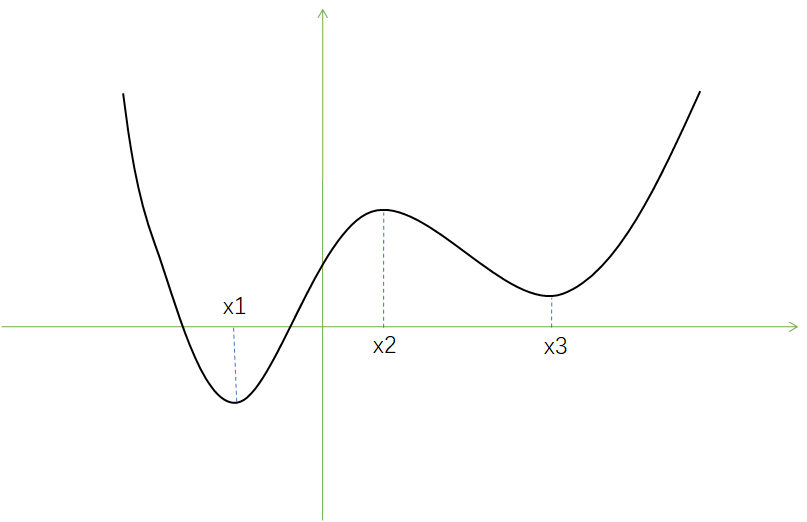经过以上的处理，我们将求解f(x)=0方程的问题转换成了求解其一阶导函数等于0的方程求解问题，这样使得我们求解的方程的次数下降了一阶。 使用这种方法，递归处理，我们最终一定能够将问题逐步转换为可以解析求解的一元二次方程。 可以看到，以上求解的过程完全不需要外部指定任何初始值以及其他变量，只需要输入方程每一项的系数，就可以求解出所有了方程实根。
代码实现（C#）
    /// <summary>
/// 求解单一未知数多项式方程，c0+c1*x^1+c2*x^2+c3*x^3+...+cn*x^n=0
/// </summary>
public class SingleUnknownPolynomialSolver
{
/// <summary>
/// 传入系数数组，构建实例
/// </summary>
/// <param name="coefs">方程系数：c0、c1、c2、c3、...、cn</param>
public SingleUnknownPolynomialSolver(params double[] coefs)
{
if (coefs == null) _c = null;
int cLength = 0;
for (int i = 0; i < coefs.Length; i++)
{
if (coefs[coefs.Length - 1 - i] == 0) continue;
cLength = coefs.Length - i;
break;
}
_c = coefs.Take(cLength).ToArray();
}

private double[] _c;
private Mathematics.ApproxEqual _approx = new Mathematics.ApproxEqual();

/// <summary>
/// 获取或设置近似相等比较器，近似相等比较器不可以为null，如果设置null则使用默认的近似相等比较器
/// </summary>
public Mathematics.ApproxEqual ApproxEqual
{
get => _approx;
set
{
if (value == null) _approx = new Mathematics.ApproxEqual();
else _approx = value;
}
}

/// <summary>
/// 求解方程的所有实数解
/// </summary>
/// <returns>返回所有实数解，无解返回空数组，无穷解返回null</returns>
public double[] Solve()
{
if (_c == null || _c.Length == 0) return null;
if (_c.Length == 1) return new double[] { };
if (_c.Length == 2) return new double[] { -_c / _c };
if (_c.Length == 3)
{
double delta = _c * _c - 4 * _c * _c;
if (delta < 0) return new double[] { };
double sqrtDelta = Math.Sqrt(delta);
if (_approx.IsEqual(sqrtDelta / _c, 0)) return new double[] { -_c / _c / 2 };
return new double[] { (-_c + sqrtDelta) / _c / 2, (-_c - sqrtDelta) / _c / 2 };
}
var partialSolver = PartialSolver();
var partialSolutions = partialSolver.Solve();
if (partialSolutions.Length == 0)
{
double? x_down = OppositePoint(0, false);
if (x_down != null)
{
double? solution = SolvePoint(x_down ?? 0, 0);
if (solution != null) return new double[] { solution ?? 0 };
else return new double[] { };
}
double? x_up = OppositePoint(0, true);
if (x_up != null)
{
double? solution = SolvePoint(0, x_up ?? 0);
if (solution != null) return new double[] { solution ?? 0 };
else return new double[] { };
}
return new double[] { };
}
List<double> solutionList = new List<double>();
var partialSolutionList = partialSolutions.ToList();
partialSolutionList.Sort();
partialSolutions = partialSolutionList.ToArray();
double? x_d = OppositePoint(partialSolutions.First(), false);
if (x_d != null)
{
double? solution = SolvePoint(x_d ?? 0, partialSolutions.First());
if (solution != null) solutionList.Add(solution ?? 0);
}
double? x_u = OppositePoint(partialSolutions.Last(), true);
if (x_u != null)
{
double? solution = SolvePoint(partialSolutions.Last(), x_u ?? 0);
if (solution != null) solutionList.Add(solution ?? 0);
}
if (partialSolutions.Length >= 2)
{
for (int i = 1; i < partialSolutions.Length; i++)
{
double? solution = SolvePoint(partialSolutions[i - 1], partialSolutions[i]);
if (solution != null) solutionList.Add(solution ?? 0);
}
}
solutionList.Sort();
for (int i = 1; i < solutionList.Count; i++)
{
if (_approx.IsEqual(solutionList[i - 1], solutionList[i]))
{
solutionList.RemoveAt(i);
i--;
}
}
return solutionList.ToArray();
}

/// <summary>
/// 计算函数值
/// </summary>
/// <param name="x"></param>
/// <returns></returns>
private double CalcValue(double x)
{
double y = 0;
for (int i = 0; i < _c.Length; i++)
{
y += _c[i] * Math.Pow(x, i);
}
return y;
}

/// <summary>
/// 一阶导函数方程
/// </summary>
/// <returns></returns>
private SingleUnknownPolynomialSolver PartialSolver()
{
double[] coefs = new double[_c.Length - 1];
for (int i = 1; i < _c.Length; i++)
{
coefs[i - 1] = _c[i] * i;
}
SingleUnknownPolynomialSolver solver = new SingleUnknownPolynomialSolver(coefs);
solver.ApproxEqual = _approx;
return solver;
}

/// <summary>
/// 求解x点函数值符号相反的点，要求指定的方向上函数是单调的
/// </summary>
/// <param name="x"></param>
/// <param name="upper">向上搜索还是向下搜索</param>
/// <returns></returns>
private double? OppositePoint(double x, bool upper = false)
{
double s = upper ? 1 : -1;
double y = CalcValue(x);
if (y == 0) return x;
double m = 1;
while (m < double.MaxValue)
{
double xp = x + m * s;
double yp = CalcValue(xp);
if (yp == 0) return xp;
if (yp == y) return null;
if (yp > y && y > 0) return null;
if (yp < y && y < 0) return null;
if (yp > 0 && y < 0) return xp;
if (xp < 0 && y > 0) return xp;
m = m * 2;
}
return null;
}

/// <summary>
/// 求解两个点中间的方程解，要求在两点之间函数单调
/// </summary>
/// <param name="xl">下方点</param>
/// <param name="xu">上方点</param>
/// <returns></returns>
private double? SolvePoint(double xl, double xu)
{
if (xl > xu) return null;
if (xl == xu)
{
if (CalcValue(xl) == 0) return xl;
else return null;
}
double l = xl;
double u = xu;
while (!_approx.IsEqual(l, u))
{
double yl = CalcValue(l);
if (yl == 0) return l;
double yu = CalcValue(u);
if (yu == 0) return u;
if (yl > 0 && yu > 0) return null;
if (yl < 0 && yu < 0) return null;
double m = (l + u) / 2;
double ym = CalcValue(m);
if (ym == 0) return m;
if (ym > 0 && yl < 0) u = m;
else if (ym > 0 && yl > 0) l = m;
else if (ym < 0 && yl < 0) l = m;
else u = m;
}
return (u + l) / 2;
}

}


关于代码的一些说明
1、算法的核心在于Solve方法中，采用嵌套调用的形式。OppositePoint方法用于处理边界区间搜索异号函数值点，SolvePoint方法用于求解在指定的区间上使用二分法求出区间上的方程根。 2、在构造函数中对输入的系数数组进行了预处理，以保证多项式的最高次项的系数不为0。 3、上述代码中没有给出Mathematics.ApproxEqual类的具体定义。实际上ApproxEqual是一个近似相等比较器，它的IsEqual方法可以比较两个实数在指定的精度和算法下是否近似相等。在实际的应用中，读者可以根据自己的需要定义自己的近似相等比较器的具体算法和参数。 4、完整的代码可以下载：https://download.csdn.net/download/weixin_45107579/13712341
展开全文算法 线性代数
• 通过对所有可能正分解的详细讨论,给出了三多项式 p-不可约的显式充要条件,该条件为由三多项式的系数构成的个简单不等式。 本文使用的主要工具是笛卡尔符号法则的推论和多项式完全判别系统相关结论等。
• 考虑到《二元齐对称多项式与二项式定理》的总结与提高，在全书的最后安排了第九章，简单介绍了个代数系统——线性空间。线性空间的基本概念，在科技领域内已可以算得上是常识性的内容(概念)了，熟悉这重要而又...
• 作者通过对实系数多项式在复数域上的分解，给出了一般非线性多项式的3周期点的等价命题。利用这等价命题，借助多项式完全判别系统，作者给出一般二映射3周期点存在性的充要条件。
• 对于分片一次多项式空间内的基函数该是怎样的呢？下面就来讲述基函数构造问题。 对于区间[x1,xn+1]上进行剖分（均匀或者非均匀剖分），然后构造如下图的分段函数： 其中Ti(x)为分段基函数，分别在区间[xi-1,xi]和...
所谓有限元就是利用有限维解决无限维问题，既然提到有限维空间，我们总能找到一组基函数的线性组合来表示这个空间内的任一元素。
对于分片一次多项式空间内的基函数该是怎样的呢？下面就来讲述基函数构造问题。
对于区间[x1,xn+1]上进行剖分（均匀或者非均匀剖分），然后构造如下图的分段函数：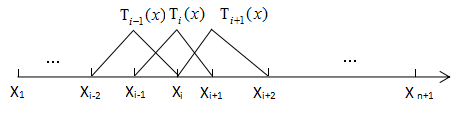其中Ti(x)为分段基函数，分别在区间[xi-1,xi]和[xi,xi+1]上都为一次函数.
其中，基函数分段函数表达形式如下：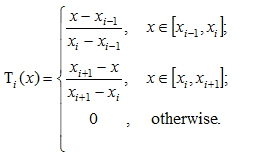有兴趣的同学可以证明这组基函数是线性无关的，并且可以线性张成这个有限维分片一次多项式空间，不妨记这个空间为Vh,无限维的分片一次多项式空间V，可以看出Vh是V的子空间。对于任意uh(x)在Vh空间中，uh(x)都可以被{Ti(x)}线性表示，不妨记为如下：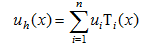其中ui是常数.我们利用上述基函数来解决如下泊松问题：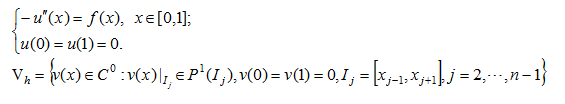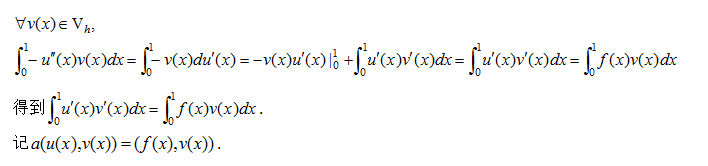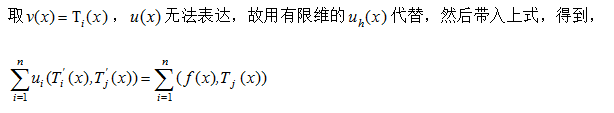解上述线性方程组即可.为了求解方便，我们采用均匀剖分的情况，当然非均匀的采用遍历法即可。假设xi+1-xi=h(固定常数)。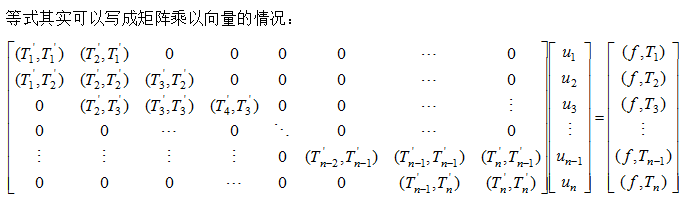现在的问题就是如何算这些内积，因为基函数是分段的，所以必须依赖于它们各自的区间进行。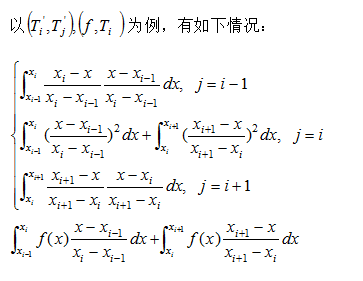注意，一个基函数最多与左右相邻的基函数有关系，其它的基函数在此区间上都为0，所以不予考虑；这样就能够把上述线性方程组求解出来，对于不同的n逼近效果不同，如果n(程序里我用的N表示)取得足够大，那么逼近效果将会非常好。下面我给出如下泊松方程的逼近情况: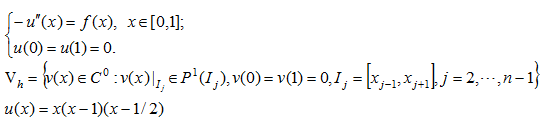逼近效果如下图：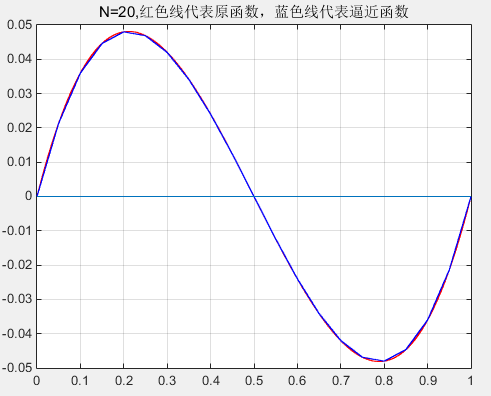可以看出当N=20的情况下逼近效果已经非常好了，这也说明有限元的做法确实可行，那么逼近过程中误差的收敛的情况如何呢？收敛阶是多少呢？如果学过有限元逼近理论应该知道，对于分片一次多项式逼近，其误差收敛阶在2阶左右（不同的误差度量，误差结果不一样，收敛阶也不完全相同，但都在2阶附近），感兴趣的同学可以自己去编程测试.
至此，分片一次多项式空间的逼近理论就暂时讲到这。不懂得请留言评论……


展开全文• 对于一元多项式，我们完全可以利用线性表P(a0,a1,a2,…,an)表示，这样的线性表在求两个多项式相加等操作时确实简单，但是多于如下的多项式： 利用上述线性表将存储为S(1,0,0,0,0,…,3,…,2);这样的结构显然对内存...
数据结构之链表创建一元多项式，求一元多项式之和
前言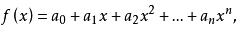对于一元多项式，我们完全可以利用线性表P(a0,a1,a2,…,an)表示，这样的线性表在求两个多项式相加等操作时确实简单，但是多于如下的多项式：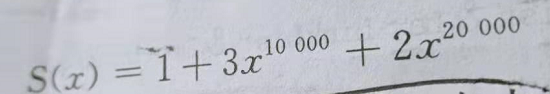利用上述线性表将存储为S(1,0,0,0,0,…,3,…,2);这样的结构显然对内存造成浪费 为了解决以上问题，可以将上面的线性表改善为P((a1,e1),(a2,e2),…,(am,em))只存储系数不为0的项，下面是该线性表的链式存储结构的代码实现。
#include "stdafx.h"
#include "stdio.h"
#include "malloc.h"
#include "stdlib.h"

#define LEN sizeof(node);

typedef struct polynode
{
int coef;//系数
int exp;//指数
struct polynode *next;
}node,*ListPolynode;

/*倒序创建一元多项式*/
void createPoly(ListPolynode L,int n)
{
ListPolynode p;
for (int i=n;i>0;i--)
{
p=(ListPolynode)malloc(sizeof(node));
printf("请输入第%d项系数\n",i);
scanf_s("%d",&p->coef);
printf("请输入第%d项指数\n",i);
scanf_s("%d",&p->exp);
p->next=L->next;
L->next=p;
}
}
/*正序创建一元多项式*/
ListPolynode createPoly1(ListPolynode L,int n)
{
ListPolynode p;
for (int i=0;i<n;i++)
{
p=(ListPolynode)malloc(sizeof(node));
printf("请输入第%d项系数\n",i+1);
scanf_s("%d",&p->coef);
printf("请输入第%d项指数\n",i+1);
scanf_s("%d",&p->exp);
L->next=p;
p->next=NULL;
L=L->next;
}
}
/*打印一元多项式*/
void printfPoly(ListPolynode L)
{
ListPolynode p=L->next;
while(p)
{
printf("%d*x^%d ",p->coef,p->exp);
p=p->next;
}
printf("\n");
}

/*多项式相加*/
{
ListPolynode currenLa=La->next;//指向La的当前结点
ListPolynode currenLb=Lb->next;//指向Lb的当前结点
ListPolynode temp;//待释放空间的临时结点
ListPolynode pre=La;/*位置指针，指向和多项式La,利用pre修改La*/
int sum;//相同指数结点系数之和
while (currenLa!=NULL&&currenLb!=NULL)
{
if (currenLa->exp<currenLb->exp)//La的当前结点指小于于Lb的当前结点指数
{
//Lb的当前位置不变,La当前位置后移
pre->next=currenLa;
pre=pre->next;
currenLa=currenLa->next;
}
else if (currenLa->exp==currenLb->exp)//La的当前结点指数等于Lb的当前结点指数
{
sum=currenLa->coef+currenLb->coef;
if (sum!=0)
{
//将La当前结点的系数改为sum,并链接到pre；将La的当前结点后移，之后过河拆桥将La的当前结点释放
currenLa->coef=sum;
pre->next=currenLa;
pre=pre->next;
currenLa=currenLa->next;
temp=currenLb;
currenLb=currenLb->next;
free(temp);
}
else
{
temp=currenLa->next;
free(currenLa);//过河拆桥干掉La当前结点
currenLa=temp;

temp=currenLb->next;
free(currenLb);//策略雷同，过河拆桥
currenLb=temp;
}
}
else//La的当前结点指数大于Lb的当前结点指数
{
//Lb当前位置添加到La当前位置之前，同时Lb向后移动一位，La向后移动一位
pre->next=currenLb;
pre=pre->next;
currenLb=currenLb->next;
}
}

if (currenLa!=NULL)//La未结束，将其全部链接到pre
{
pre->next=currenLa;
}
else//若Lb未结束，将其全部链接到pre
{
pre->next=currenLb;
}
}

int _tmain(int argc, _TCHAR* argv[])
{
ListPolynode La=(ListPolynode)malloc(sizeof(node));
La->next=NULL;
printf("请输入创建一元多项式的项数\n");
int n;
scanf_s("%d",&n);
createPoly1(La,n);
printfPoly(La);

ListPolynode Lb=(ListPolynode)malloc(sizeof(node));
Lb->next=NULL;
printf("请输入创建一元多项式的项数\n");
scanf_s("%d",&n);
createPoly(Lb,n);
printfPoly(Lb);

printfPoly(La);

system("pause");
return 0;
}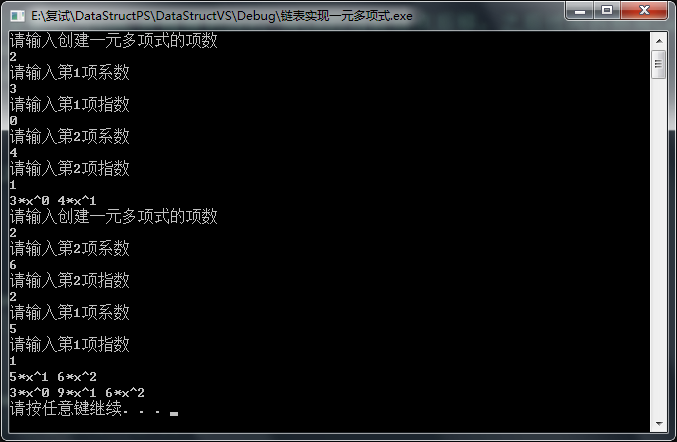展开全文数据结构
• 前言：个人实现的个用带头结点的链表实现的一元多项式运算程序。该程序支持多项式的创建、打印、加、减、乘以及取反操作。并且具备相应的用户操作菜单，用户提示菜单。其中针对多项式的各种操作已经模块化，并且每...

前言：个人实现的一个用带头结点的链表实现的一元多项式运算程序。该程序支持多项式的创建、打印、加、减、乘以及取反操作。并且具备相应的用户操作菜单，用户提示菜单。其中针对多项式的各种操作已经模块化，并且每一个函数的功能都做了非常详细的注释。话不多说，开始讲解代码。

存储结构
链表结点结构体
采用单链表的结点形式来存储多项式的每一个单项式。结构体中定义了单项式的系数和次数，以及指向下一个结点的指针。很容易理解。
typedef struct LNode
{
double coef;
int power;
struct LNode* next;
}LNode;

全局变量
全局变量定义了一个指针数组，存放指向每一个多项式的头结点的指针，并且定义了一个counter，用来记录已创建的多项式个数。
LNode* List[MaxLen];
int counter = 0;

整体框架
运行过程
主程序运行过程很简单，总体就分这三步：
输入多项式的数据选择相应的功能退出程序
是不是很简单，主要原因在于我尽量将所有的单一功能放在了一个函数中，实现了最大程度上的模块化。也就可以使得我的主程序代码尽量简单明了，仅仅做的事情就是打印用户菜单。
函数介绍
链表(多项式)复制函数
定义新指针q指向待复制链表的开始结点，定义新指针p指向新链表的头结点；创建新结点s，将q所指结点信息赋值给s，q后移；将s插入新链表；重复2、3直到q为空。
LNode* CopyPolyn(LNode* head);

链表(多项式)创建函数
定义遍历指针p指向开始结点，q指向p的前驱；循环创建新结点，将输入的数据赋值给结点数据，然后插入链表；结点按照次数从小到大的排列，所以插入链表时要做以下操作： 1）、遵循次数从小到大插入 2）、有同类项则合并 3）、合并后若为0则删除结点
void Creat(LNode* head, double iCoef, int iPower);

数据加载函数
依据用户的选择，程序执行两种数据加载函数的其中之一。其中前者为读取键盘输入，后者为读取txt文本文件输入。txt文本文件内容我会放在后文中。文本读取函数的具体执行过程用注释标注的非常详细，可以自行理解。
void CreatPolynCin();
void CreatPolynIfs();

链表选择函数
智能处理用户操作函数时输入的链表对象，既可以只操作一个链表，也可以操作多个链表。原理是借助一个数组来记录本次操作，用户希望操作的链表对象的头结点指针再全局LIst数组中的下标。
int Choose(int cho[], int len);

链表(多项式)打印函数
调用后者函数，用户输入希望打印的多项式。然后传入相应链表的头结点打印相应的多项式。同时对于输出要做一下格式化的处理。
void PrintPolyn(LNode* head);

void ChoosePrint();

链表(多项式)相加函数
LNode* AddPolyn(LNode* head1, LNode* head2);

链表(多项式)取反函数
用户选择要取反的对象，程序直接将其取反并打印输出。此函数最多取反一个函数
void Opposite(LNode* head);
void ChooseOpposite();

链表(多项式)相减函数
该函数实际上是一个减法选择函数，因为多个多项式连续相减可以转换为多个多项式连续相加。只需要将第一个多项式取反，再将最后结果取反即可得到相减的结果。
void ChooseSubtract();

链表(多项式)相乘函数
同理，用户选择两个或多个要相乘的多项式，程序会自动处理。乘法的原理也很简单，同样的能化成加法来操作。在这里，我采用的是传统的，即我们平常笔算时用到的多项式乘法规则——项项相乘再相加。感兴趣的同学可以关注我的另一篇博客FFT应用于多项式乘法。这篇博客介绍了如何加快多项式乘法的算法，尤其在面对项数特别大时的情况，有着非常好的优化效果。但是对于项数一千以内的多项式乘法，逐个相乘的效果是要好一点的。
LNode* MutPolyn(LNode* head1, LNode* head2);
void ChooseMutiply();

txt文本文件的内容

1.9 2 2 5 5 2 2.5 4 -1.5 2 -2 5 2 4 5 2 5.8 2 -2 4 8 9 5 2 -1 2 -2 5

其实这个你们完全可以自己写的，但是为了方便还是把我自己的，比较完整的测试数据放在这里，请自行取用吧。
完整代码
#include <cmath>
#include <cstdio>
#include <cstdlib>
#include <fstream>
#include <iostream>
#include <windows.h>
#define MaxLen 1024
using namespace std;

typedef struct LNode // 定义结点结构体
{
double coef; // coefficient系数
int power; // 次数
struct LNode* next;
}LNode;
LNode* List[MaxLen]; // 结点指针数组，存放多项式的头指针
int counter = 0; // 全局计数器，表示已存在多项式个数

// 链表的复制
{
/*
*/
LNode* q = head->next; // 一定要给下一个结点，否则头结点也会被复制进去

while (q != NULL)
{
LNode* s = new LNode;
s->coef = q->coef;
s->power = q->power;
p->next = s;
p = s;
q = q->next;
}
p->next = NULL;
}

//创建结点的函数，提高可重用性
void Creat(LNode* head, double iCoef, int iPower)
{
LNode* p = head->next; // p是遍历指针
LNode* q = head; // q是p的前驱指针

while (1) // 该循环比较p指向的项的次数和输入次数的大小来判断操作
{
if (p == NULL || p->power > iPower)
{
/*
如果找到了比输入次数大的项，就新建一个结点插在该项之前
或者p指向了空，说明不存在比输入次数大的项，就在表尾插入新结点
p == NULL放在第一个if判断，否则在分支判断中会因为读取不到数据出问题
而且由短路逻辑可知，p == NULL判断为真后就不再判断后一个，同样不会出问题
*/
p = new LNode; // 直接令p指向一个新结点
p->coef = iCoef;
p->power = iPower;
p->next = q->next;
q->next = p;
break; // 退出循环，读取下一个输入
}
else if (p->power < iPower)
{ // 如果输入的次数更大则往后比较下一个结点
p = p->next;
q = q->next;
}
else if (p->power == iPower) // 如果次数一样就合并同类项
{
if (p->coef + iCoef == 0) // 如果系数相反就删除结点
{
q->next = p->next;
free(p); // 释放结点p的空间
p = q->next; // 继续让p指向q的后继，为空的话直接退出循环
}
else
p->coef += iCoef;
break; // 上述无论哪个操作执行完都要退出循环读取下一个输入
}
}
}

// 手动输入创建链表
void CreatPolynCin()
{
int inputPower; // 输入指数
double inputCoef; // 输入系数

LNode* head = new LNode; // 创建头结点

cin.get(); // 过滤回车
printf("\t请按照“系数”“次数”的格式输入多项式的各项\n\t");

while (cin.peek() != EOF && cin.peek() != '\n') // 读到文件结尾或者回车时退出循环，按次数从小到大插入新的结点
{
cin >> inputCoef >> inputPower;
}
printf("\t成功创建了第%d个多项式\n\n", counter);
}
// 文本输入创建链表
void CreatPolynIfs()
{
int i = 0, j, len, flag; // flag标记是否为负数
double temp; // temp存储读到的数
double input[MaxLen]; // input数组保存多项式的项
char line[MaxLen]; // line字符串保存

printf("\t正在读取文件，请稍等：\n");
printf("\t加载中：");
while ((i += 10) < 100)
{
Sleep(300);
printf("*");
}
printf("加载完成！\n");
Sleep(1000);

ifstream file; // 定义输入文本
file.open("file.txt", ios::in); // 以读方式打开

while (file.getline(line, MaxLen)) // 逐行读取最大MaxLen个字符，读取到EOF返回-1
{
for (i = 0, len = 0; line[i] != '\0'; i++)
{
temp = 0, flag = 0;
if (line[i] >= '0' && line[i] <= '9')
{
if (line[i - 1] == '-') // 前面有负号的话标记一下
flag = 1;
for (j = i; line[j] != '\0' && line[j] != '.' && line[j] != ' '; j++) // 开始循环找完整的一个数
{ // 循环结束的条件是读到了字符串的终止符或读到了小数点或读到了空格
temp = temp * 10 + line[j] - '0';
}
if (line[j] == ' ') // 读到空格说明该数读完了，把j值赋给i跳过数字占用的字符
i = j - 1;
if (line[j] == '.') // 如果读到了小数点
{
double num = 0, point = 0; // num记录小数部分，point记录小数数位
for (j += 1; line[j] != '\0' && line[j] != ' '; j++) // j += 1表示挪到第一位小数
{ // 由于已经读到了小数点，所以循环结束的条件是读到了字符串的终止符或读到了空格
num = num * 10 + line[j] - '0';
point++;
}
temp += num / pow(10, point); // 将num转为小数加到temp上
i = j - 1;
}
flag ? input[len++] = -1 * temp : input[len++] = temp;
}
}

for (i = 0; i < len; i += 2) // 每两个相邻元素取出来作为一个项创建新结点
Creat(head, input[i], (int)input[i + 1]); // 别忘记转换类型，不用担心丢失精度，次数是整数
printf("\t成功创建了第%d个多项式\n\n", counter);
}
}

// 选择要操作的链表
int Choose(int cho[], int len)
{
while (cin.peek() != '\n')
{
cin >> cho[len];
if (cho[len] <= 0 || cho[len] > counter)
{
printf("\t您输入了不存在的%d号多项式！\n", cho[len]);
cin.sync(); // 如果不存在的多项式后仍然有输入，该函数将清空它们以免读取进了用户操作
return 0;
}
else
++len;
}
return len;
}

// 多项式打印
{
while (p != NULL)
{
// 因为次数递增，因此次数为0的情况只可能发生在第一个，后面就无需判断次数是否为0
if (p->power == 0) // 常数项直接输出
printf("\t%.1lf", p->coef);
else
{
if (p->coef > 0 && p != head->next) // 如果系数大于0且不是首项
printf("+%.1lfx^%d", p->coef, p->power);
else if (p->coef < 0 && p != head->next) // 如果系数小于0且不是首项
printf("%.1lfx^%d", p->coef, p->power);
else // 如果是首项直接输出
printf("\t%.1lfx^%d", p->coef, p->power);
}
p = p->next;
}
printf("\n\n");
}
// 用户选择要打印的多项式
void ChoosePrint()
{
int snum[MaxLen] = { 0 }; // serial number序号
int serNum = 0;
cin.get();
printf("\t请输入您要打印的多项式的序号\n\t");
serNum = Choose(snum, serNum);

for (int i = 0; i < serNum; i++)
{
printf("\t第%d个多项式是:", snum[i]);
PrintPolyn(List[snum[i]]);
}
if (!serNum) return;
}

// 多项式相加
{
/*
加法原理：
*/

while (p != NULL)
{
p = p->next;
}
}
// 用户选择要相加的多项式
{
int add[MaxLen] = { 0 };
int addNum = 0; // 计录参与相加的多项式个数，标记是否输入错误的标志

cin.get();
printf("\t请输入参与相加的多项式序号\n\t");

for (int i = 1; i < addNum; i++) // 逐个相加
/*
如果相加后的结果只有一个常数，就要存入一个系数次数都为0的结点
而创建结点和相加函数要经常调用，因此不适合在它们内部放入这个操作
*/
{
LNode* s = new LNode;
s->coef = 0;
s->power = 0;
s->next = NULL;
}
printf("\t相加后的结果是(第%d个多项式)：\n", counter);
}

// 多项式取反
{
while (p != NULL)
{
p->coef *= -1;
p = p->next;
}
}
// 用户选择取反的多项式
void ChooseOpposite()
{
int oppoNum;
printf("\t请输入要取反的多项式序号\n\t");
cin >> oppoNum;
if (oppoNum > counter || oppoNum <= 0)
printf("\t您要取反的多项式不存在!\n");
else
{
Opposite(List[oppoNum]);
printf("\t取反后的结果是\n");
PrintPolyn(List[oppoNum]);
}
}

// 用户选择要相减的多项式并执行多项相减
void ChooseSubtract()
{
int sub[MaxLen] = { 0 };
int subNum = 0, flag = 0; // 计录参与相加的多项式的个数，标记是否输入错误的标志

cin.get();
printf("\t请输入参与相减的多项式序号\n\t");
subNum = Choose(sub, subNum);
if (!subNum) // 返回0表明输入序号异常，退出该操作
return;

for (int i = 1; i < subNum; i++) // 逐个相加

{
LNode* s = new LNode;
s->coef = 0;
s->power = 0;
s->next = NULL;
}
printf("\t相减后的结果是(第%d个多项式)：\n", counter);
}

// 多项式相乘
{
while (p != NULL)
{
while (q != NULL)
{
Creat(head, p->coef * q->coef, p->power + q->power);
q = q->next;
}
p = p->next;
}
}
// 用户选择要相乘的多项式
void ChooseMutiply()
{
int mut = { 0 };
int mutNum = 0; // 计录参与相乘的多项式个数

cin.get();
cout << "\t请输入参与相乘的多项式序号\n\t";
mutNum = Choose(mut, mutNum);
if (!mutNum) return;

LNode* mutHead = List[mut]; // 新建好和式链表头结点并初始化以便参与递归
for (int i = 1; i < mutNum; i++) // 逐个相加

cout << "\t相加后的结果是(第" << counter << "个多项式)：" << endl;
}

// 根据用户选择执行操作
void Operation(int choice)
{
switch (choice)
{
case 1:
printf("\t请问您要手动输入还是文本输入？\n");
printf("\t1.我不怕累！我要手动输入！\n");
printf("\t2.手太酸了，文本输入吧...\n\t");
int c;
cin >> c;
if (c == 1)
CreatPolynCin();
else
CreatPolynIfs();
break;
case 2:
ChoosePrint();
break;
case 3:
break;
case 4:
ChooseSubtract();
break;
case 5:
ChooseMutiply();
break;
case 6:
ChooseOpposite();
break;
case 7:
printf("\t又在暗示我厂，7777777！\n");
exit(0);
case 8:
printf("\t我就知道您会瞎选，嘿嘿\n");
break;
default:
printf("\t抱歉该选项功能还未开发！请重新输入！\n");
break;
}
}

// 主函数入口
int main(void)
{
int choice;
printf("\t\t程序初始化成功!\n");
printf("\t\t  欢迎Ripe王!\n");

while (1)
{
printf("\t*******************************\n");
printf("\t***  请选择您要执行的操作   ***\n");
printf("\t***      1.创建多项式       ***\n");
printf("\t***      2.打印多项式       ***\n");
printf("\t***      3.多项式相加       ***\n");
printf("\t***      4.多项式相减       ***\n");
printf("\t***      5.多项式相乘       ***\n");
printf("\t***      6.多项式取反       ***\n");
printf("\t***       7.退出程序        ***\n");
printf("\t*******************************\n");
printf("\t");
cin >> choice;
Operation(choice);
}
return 0;
}


希望本篇博客能对你起到一点的帮助作用，也希望能动动小手点个赞，这样我才能知道，我的付出没有白费啦~~。
展开全文链表 数据结构
• f(x)是GF(q)上个无平方因式的n次多项式，(0)≠O。Nq,k表示GF(q)中使，f (ζ)是个K次方幂的元根ζ的个数。本文研究Nq,k的下界。例如，我们证明了，如果√q≥n(k-1)2ω(q-1)，则Nq,k>0，这里}c>1，ω(m)表m的不同...
• 本文使用 Zhihu On VSCode 创作并发布0 前言...N 元一次线性回归问题，并基于线性代数 C++ 模板库——Eigen 进行了实现，最后，比较了几种实现方法在求解速度与求解精度上的差异。1 最小二乘法概述最小二乘法（Lea...
• 题目说明： ...对应一组输入，输出一次操作的结果（参见测试用例）。 1 多项式输出格式：以指数递增的顺序输出: <系数,指数>,<系数,指数>,<系数,指数>,参见测试用例。零多项式的输出格式为,0> 0 无输出
• 文章目录多项式回归多项式回归二、scikit-learn中的多项式回归三、关于PolynomialFeatures四、sklearn中的Pipeline五、过拟合和欠拟合六、解决过拟合问题七、透过学习曲线看过拟合我是尾巴 直线回归研究的是...机器学习
• 一元多项式乘法算法    一般的，一元多项式相乘有两种算法： ...算法：结果用链表存储 此算法用A（i）去乘B（j）(0&lt;=j&lt;m)，逐项把每个结果插入结果链表ResultNode中。此算...算法 C C++ C#
• 多项式均表示为数组形式，数组元素为多项式降幂系数 1. polyval函数 求多项式在某一点或某几个点的值. p = [1,1,1];%x^2+x+1 x = [-1,0,1];y = polyval(p,x); 另外求函数在某一点或某几个点的值可以用函数feval. x ...MATLAB
• 一元稀疏多项式计算器班级：02计2 姓名：刘晓明 学号：200201020219完成日期：2004年9月30日 ，需求分析： 1， 输入并建立多项式2， 输出多项式3， 相同指数的同类项合并4， 对于没有指数的项，把指数设为...null iostream struct c list
•C语言 PAT
• 线性递推可以用多项式取模优化做到O(mlogmlogn)的优秀复杂度。本文主要介绍了不借助矩阵来理解多项式取模与线性递推关系的巧妙方法
• 数据结构与算法分析——c语言描述 ...这里完全是来个二循环排序，应该有更好的办法，而且排序嫌麻烦直接交换内容而不是用了链表的交换。以后会更新代码的。 list.h typedef struct { int Coefficient; int Expone
• 所以我们可以简单的认为任何个正整数都可以用2的n幂来表示。事实上我们可以进一步看这个问题：如何把个十进制数转化为二进制数。一般的方法是把个十进制数不断除以2，直到商为零，依次写下余数。所以开始的...算法 c++
• ## 多项式的各种操作

千次阅读 多人点赞 2017-06-14 19:31:29
多项式的各种操作By SemiWaker 多项式逆元 多项式除法 多项式牛顿迭代法 多项式对数 多项式指数函数 多项式幂函数 多项式三角函数 多项式多点求值 多项式快速插值
• 多项式加法实现原理： （1）如果cpa的指数<cpb的指数，则将cpa所指向的插入和多项式链中，向后移动指针； （2）如果cpa的指数>cpb的指数，则将cpb所指向的插入和多项式链中，向后移动指针； （3）如果cpa的...数据结构 算法
• 　从给定的函数表出发，寻找个简单合理的函数近似表达式来拟合给定的组数据。 这里所说的“拟合”,即不要所作的曲线完全通过所有的Σ数据点，只要求所得的近似曲线能反映数据的基本趋势。数据拟合在实际中...java 单链表
• 1010 一元多项式求导 （25 分) 设计函数求一元多项式的导数。（注：x​n​​（n为整数）的一阶导数为nx​n−1​​。） 输入格式: 以指数递降方式输入多项式非零项系数和指数（绝对值均为不超过 1000 的整数）。...
• 这个题目麻烦的地方在于考虑输出格式。比较训练对函数模块的抽象能力，好的抽象可以保证不出现大堆if语句。个比较关键的点是，当两个多项式的和/差为0时，应当输出0，这是很多代码不被AC的原因。c语言 c++
• ## matlab多项式操作

千次阅读 2016-08-31 02:01:28
实例：设计个函数 poly2str()，实现把个行向量表示的多项式转换为常见的字符串表 示的多项式， %poly2str.m %把多项式的行向量表示转换为字符串表示 function Y=poly2str(X) %X 是表示多项式的向量 %Y ...
• 一元多项式计算器 (c语言数据结构实验） 班级 ： **** 学号 ： 201907020633 姓名 ： *** 实验机号: A3 实验日期 :2020.12.04 报告日期 :2020.12.07 实验题目：一元多项式计算器算法 数据结构
• 考虑下面的数据，虽然我们可以使用线性回归来拟合这些数据，但是这些数据更像是条二曲线，相应的方程是y=ax2+bx+c，这个式子虽然可以理解为二方程，但是我们呢可以从另外个角度来理解这个式子： 如果...机器学习算法...